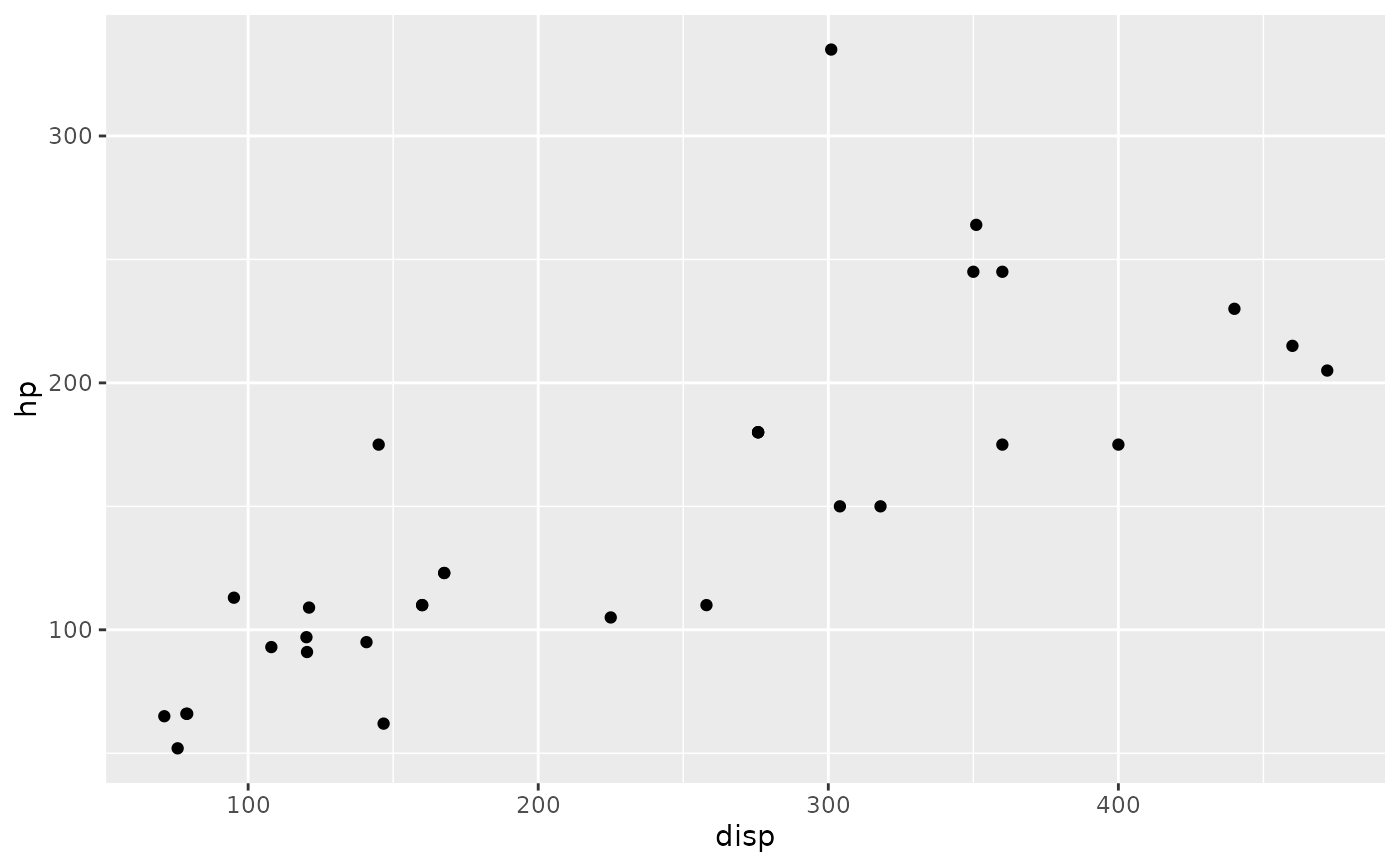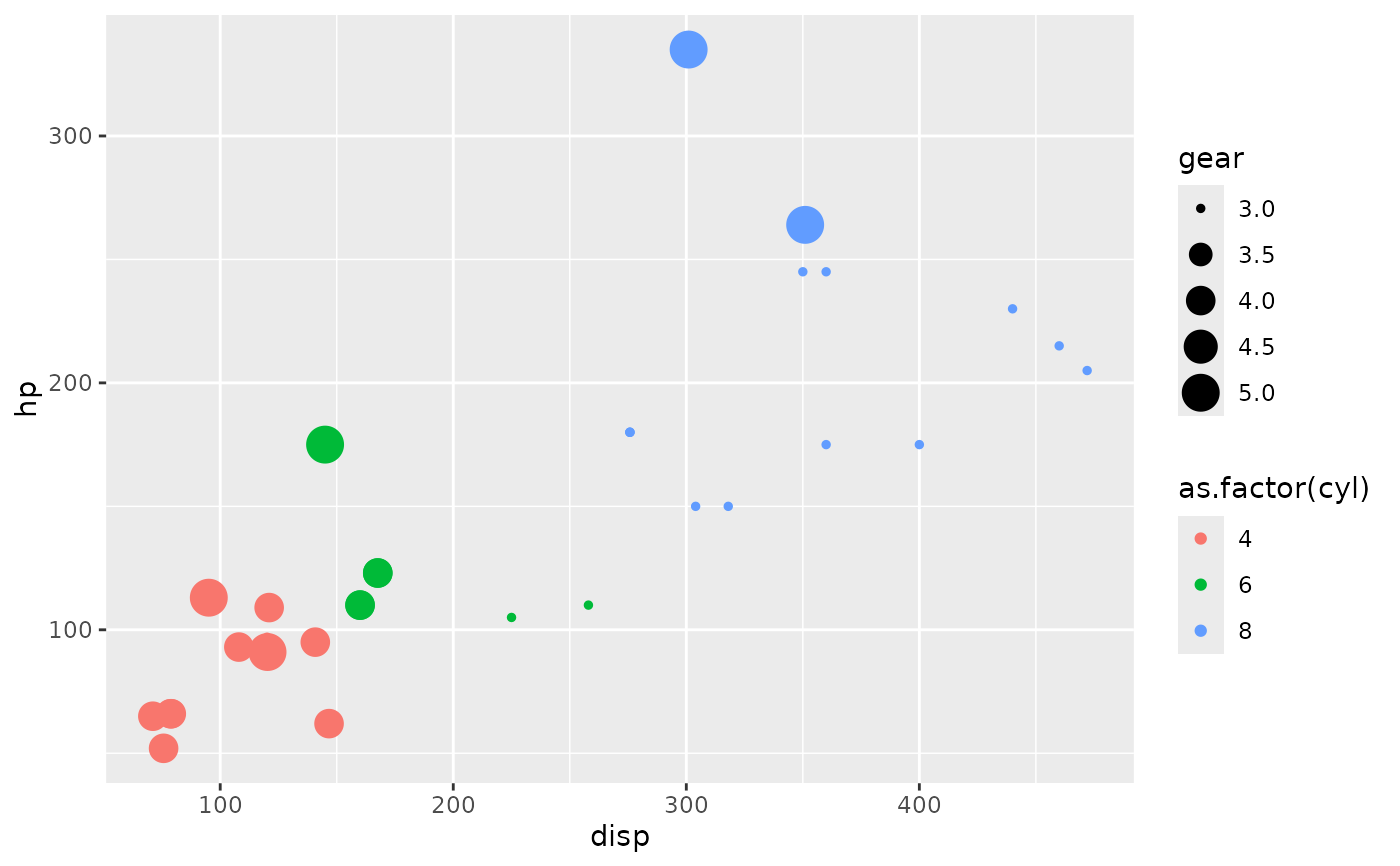Make a scatter plot with a given data set.

ggally_points(data, mapping, ...)

## Arguments

data data set using aesthetics being used other arguments are sent to geom_point

Barret Schloerke

## Examples

# Small function to display plots only if it's interactive
p_ <- GGally::print_if_interactive

data(mtcars)
p_(ggally_points(mtcars, mapping = ggplot2::aes(x = disp, y = hp)))p_(ggally_points(mtcars, mapping = ggplot2::aes_string(x = "disp", y = "hp")))p_(ggally_points(
mtcars,
mapping = ggplot2::aes_string(
x     = "disp",
y     = "hp",
color = "as.factor(cyl)",
size  = "gear"
)
))